## 20160226

### Presentation: diffraction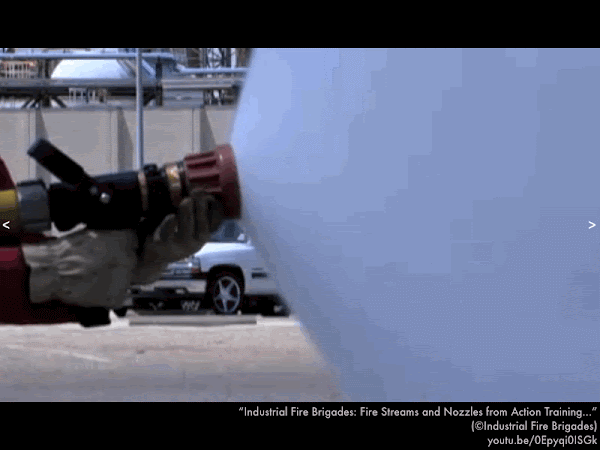Look at this fire hose nozzle. Just look at it. Pinching the flow of water makes it spread out more; while opening up the nozzle narrows the spread of water.

This is by no means explains what we will see with waves that move through a single opening, but this very crude analogy will serve our purposes well enough.Previously we considered the interference of waves from separate in phase sources, as monochromatic (same wavelength λ light) through two slits. Here we look at diffraction, which is the spread of light from a single slit.First, terminology.Notice how these parallel water wavefronts spread out after passing through the central opening in this inlet. This is an example of how waves diffract as they pass through a "single-slit" opening.The relevant parameters are the wavelength λ of the parallel wavefronts and the slit opening width W, which affect how these waves diffract and spread out, given by the "half-angle" θ, as measured from the center line.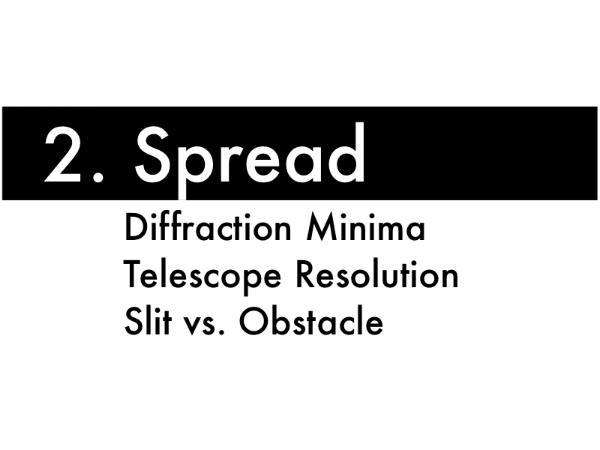Second, quantifying the spread of these waves after diffracting through the singe slit.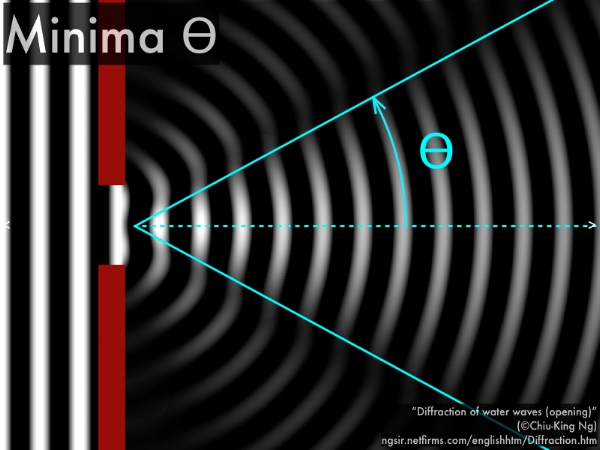If you squint (in order to increase the contrast of the diffracted wavefronts), you can make out a faint destructive region on either side of the center line, which forms the boundary of most of the diffracted wave energy. This is the first minima angle θ.The equation for this diffraction minima is given by Wsinθ = mλ, where θ is the "half-angle" of the spread of the diffracted waves, and m = 1 (which if this doesn't freak you out by the resemblance to the double-slit maxima equation, it should). Just work with this, and let's see what it can tell us.Since the slit width W and spread half-angle θ appear on both sides of the first diffraction minima equation, then making the slit opening smaller would result in increasing the spread of the diffracted waves.Also making the slit opening larger would result in decreasing the spread of the diffracted waves.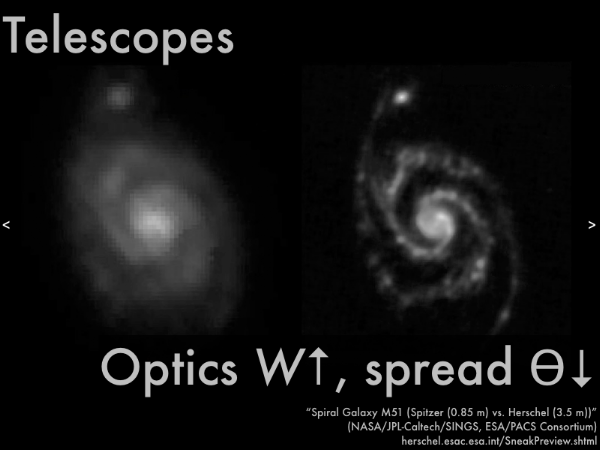An example of this is the diffraction of light through the circular aperture of a telescope with a "width" W (although the more correct equation in this case would have a correction for a diameter of a circular opening: Wsinθ = (1.22)(1)λ). This is the Whirlpool Galaxy M51 as seen by the NASA Spitzer Space Telescope and the European Space Agency Herschel Space Telescope, as observed with the same infrared wavelength λ. As the Spitzer Space Telescope has a much smaller mirror diameter, light from each part of the galaxy will diffract more and spread out more, resulting in a much less resolved image than from the Herschel Space Telescope, with a much larger mirror diameter, such that light from each part of the galaxy will diffract less and spread out less, resulting in a much better resolved image with finer details left intact.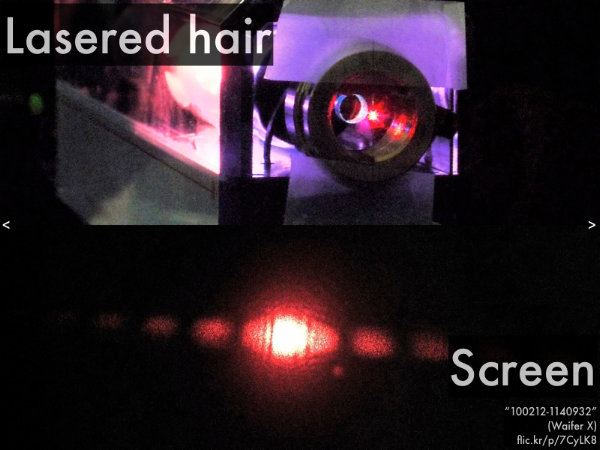In laboratory you will shine a laser on your hair. Since the lasers are relatively low-powered (but don't shine them in your eyes), you won't be able to burn through your hairs, but light will diffract around either side of the hair shaft. This turns out to be entirely equivalent to light shining through a single slit of the same width as your hair, and the resulting diffraction pattern on a distant screen shows the spread of light contained within the first minima θ angles on either side of the center line. Depending on how thick your hair is will determine how little (or much) light will diffract and spread out on the screen.